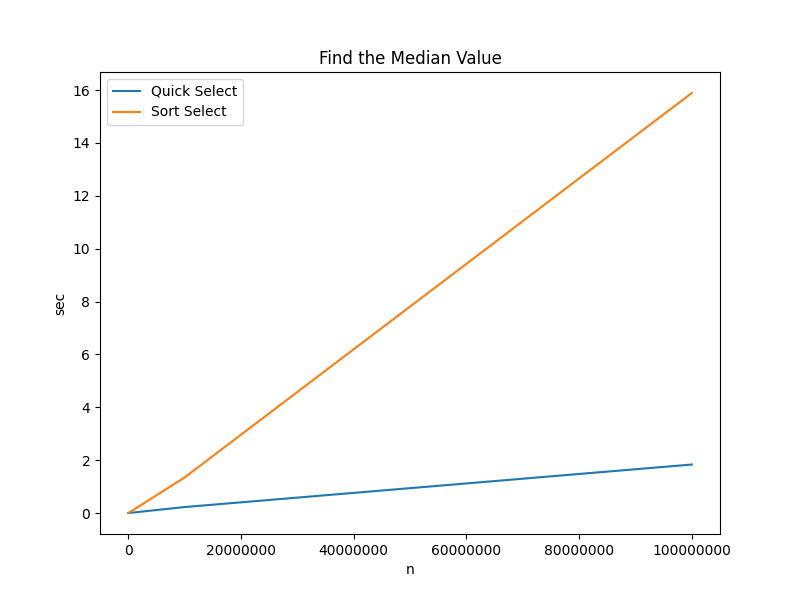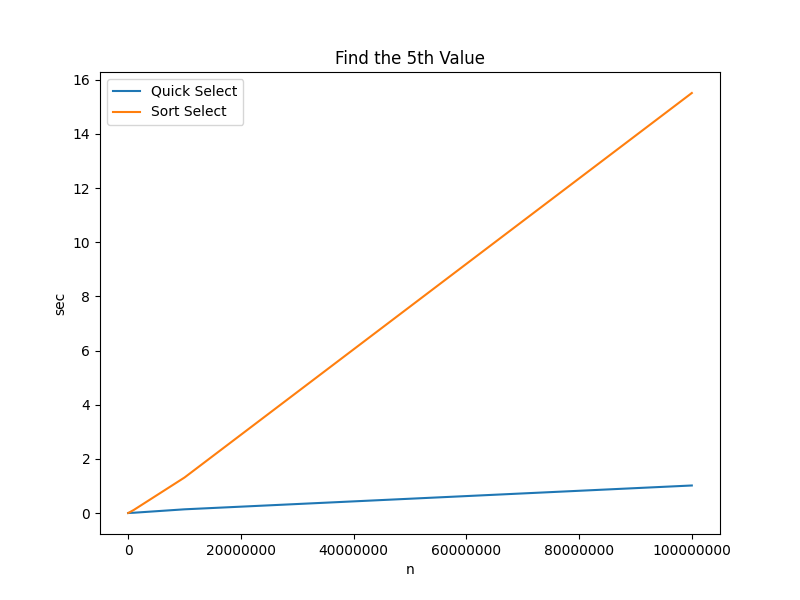The actual run times for finding the median1, 5th highest, and minimum value in an integer array using the C implementation of the quick select algorithm and C’s qsort are shown below.

## Find the Median ValueALGORITHM n=100 n=1000 n=10000 n=100000 n=1000000
QUICK_SELECT 0.000002 sec 0.000038 sec 0.000443 sec 0.002946 sec 0.042520 sec
SORT_SELECT 0.000006 sec 0.000096 sec 0.000704 sec 0.008697 sec 0.104405 sec

## Find the 5th ValueALGORITHM n=100 n=1000 n=10000 n=100000 n=1000000
QUICK_SELECT 0.000006 sec 0.000011 sec 0.000074 sec 0.001099 sec 0.009004 sec
SORT_SELECT 0.000004 sec 0.000082 sec 0.000707 sec 0.008884 sec 0.104003 sec

## Find the Minimum Value

For the maximum/minimum value in an array, it’s possible to do a simple linear scan while keeping track of the highest/lowest value. The result is shown below.ALGORITHM n=100 n=1000 n=10000 n=100000 n=1000000
QUICK_SELECT 0.000003 sec 0.000010 sec 0.000468 sec 0.002036 sec 0.023628 sec
SORT_SELECT 0.000005 sec 0.000056 sec 0.000814 sec 0.008738 sec 0.103920 sec
LINEAR_SCAN_MIN 0.000001 sec 0.000002 sec 0.000030 sec 0.000143 sec 0.001475 sec

Key Takeaway:

• Quick Select and Linear Scan are both $O(n)$ algorithms. Obviously, they do not have the same actual run times. It’s important to understand that the purpose of asymptotic time complexity is to demonstrate how the run time of an algorithm increases as the input size increases. It is not the only run time consideration.

## Source Code

Full Repo

Relevant Files:

1. For the purposes of this project, median is defined as simply the $n/2$th element. Technically, if the array is odd sized, it should be the average of the middle two elements. A somewhat pedantic point, but it may be important in some contexts.